## NAME

r.green.hydro.financial - Assess the financial costs and values

## SYNOPSIS

r.green.hydro.financial
r.green.hydro.financial --help
r.green.hydro.financial plant=name struct=name [plant_layer=string] [struct_layer=string] [struct_column_id=string] [struct_column_power=string] [struct_column_head=string] [struct_column_side=string] [struct_column_kind=string] [struct_kind_intake=string] [struct_kind_turbine=string] [plant_column_id=string] [plant_basename=string] [interest_rate=float] [gamma_comp=float] [life=float] [landvalue=name] [tributes=name] [stumpage=name] [rotation=name] [age=name] [landuse=name] [rules_landvalue=string] [rules_tributes=string] [rules_stumpage=string] [rules_rotation=string] [rules_age=string] [width=float] [depth=float] [slope_limit=float] [min_exc=name] [max_exc=name] slope=name [rules_min_exc=string] [rules_max_exc=string] [alpha_em=float] [beta_em=float] [gamma_em=float] [const_em=float] [lc_pipe=float] [lc_electro=float] electro=name [electro_layer=string] [elines=string] [alpha_station=float] [alpha_inlet=float] [grid=float] [general=float] [hindrances=float] [cost_maintenance_per_kw=float] [alpha_maintenance=float] [beta_maintenance=float] [const_maintenance=float] [energy_price=float] [eta=float] [operative_hours=float] [const_revenue=float] output_struct=name [compensation=name] [excavation=name] [upper=name] [--overwrite] [--help] [--verbose] [--quiet] [--ui]

### Flags:

--overwrite
Allow output files to overwrite existing files
--help
Print usage summary
--verbose
Verbose module output
--quiet
Quiet module output
--ui
Force launching GUI dialog

### Parameters:

plant=name [required]
Name of the input vector map with the segments of the plants
Or data source for direct OGR access
struct=name [required]
Name of the input vector map with the structure of the plants
Or data source for direct OGR access
plant_layer=string
Name of the vector map layer of the segments
Vector features can have category values in different layers. This number determines which layer to use. When used with direct OGR access this is the layer name.
Default: 1
struct_layer=string
Name of the vector map layer of the structure of the plants
Vector features can have category values in different layers. This number determines which layer to use. When used with direct OGR access this is the layer name.
Default: 1
struct_column_id=string
Table of the struct map: column name with plant id
Default: plant_id
struct_column_power=string
Table of the struct map: column name with power value
Default: power
Table of the struct map: column name with head value
struct_column_side=string
Table of the struct map: column name with the strings that define the side of the plant
Default: side
struct_column_kind=string
Table of the struct map: column name with the strings that define if it's a derivation channel or a penstock
Default: kind
struct_kind_intake=string
Table of the structures map: Value contained in the column 'kind' which corresponds to the derivation channel
Default: conduct
struct_kind_turbine=string
Table of the structures map: Value contained in the column 'kind' which corresponds to the penstock
Default: penstock
plant_column_id=string
Table of the plants map: Column name with the plant id
Default: plant_id
plant_basename=string
Table of the plants map: basename of the columns that will be added to the input plants vector map
Default: case1
interest_rate=float
Interest rate value
Default: 0.03
gamma_comp=float
Coefficient
Default: 1.25
life=float
Life of the hydropower plant [year]
Default: 30
landvalue=name
Name of the raster map with the land value [currency/ha]
Name of input raster map
tributes=name
Name of the raster map with the tributes [currency/ha]
Name of input raster map
stumpage=name
Name of the raster map with the stumpage value [currency/ha]
Name of input raster map
rotation=name
Name of the raster map with the rotation period per landuse type [year]
Name of input raster map
age=name
Name of the raster map with the average age [year]
Name of input raster map
landuse=name
Name of the raster map with the landuse categories
Name of input raster map
rules_landvalue=string
Rule file for the reclassification of the landuse to associate a value for each landuse [currency/ha]
rules_tributes=string
Rule file for the reclassification of the landuse to associate a tribute rate for each landuse [currency/ha]
rules_stumpage=string
Rule file for the reclassification of the landuse to associate a stumpage value for each landuse [currency/ha]
rules_rotation=string
Rule file for the reclassification of the landuse to associate a rotation value for each landuse [year]
rules_age=string
Rule file for the reclassification of the landuse to associate an age for each landuse [year]
width=float
Width of the excavation works [m]
Default: 2.
depth=float
Depth of the excavation works [m]
Default: 2.
slope_limit=float
Slope limit, above this limit the cost will be equal to the maximum [degree]
Default: 50.
min_exc=name
Minimum excavation costs [currency/mc]
Name of input raster map
max_exc=name
Maximum excavation costs [currency/mc]
Name of input raster map
slope=name [required]
Slope raster map
Name of input raster map
rules_min_exc=string
Rule file for the reclassification of the landuse to associate a minimum excavation cost for each landuse [currency/mc].
rules_max_exc=string
Rule file for the reclassification of the landuse to associate a maximum excavation cost for each landuse [currency/mc].
alpha_em=float
Electro-mechanical costs alpha parameter, default values taken from Aggidis et al. 2010
Default: 0.56
beta_em=float
Electro-mechanical costs beta parameter, default values taken from Aggidis et al. 2010
Default: -0.112
gamma_em=float
Electro-mechanical costs gamma parameter, default values taken from Aggidis et al. 2010
Default: 15600.0
const_em=float
Electro-mechanical costs constant value, default values taken from Aggidis et al. 2010
Default: 0.
lc_pipe=float
Supply and installation linear cost for the pipeline [currency/m]
Default: 310.
lc_electro=float
Supply and installation linear cost for the electroline [currency/m]
Default: 250.
electro=name [required]
Name of the vector map with the electric grid
Or data source for direct OGR access
electro_layer=string
Vector map layer of the grid
Default: 1
elines=string
Output name of the vector map with power lines
alpha_station=float
Power station costs are assessed as a fraction of the Electro-mechanical costs
Default: 0.52
alpha_inlet=float
Inlet costs are assessed as a fraction of the Electro-mechanical costs
Default: 0.38
grid=float
Cost for grid connection
Default: 50000
general=float
Factor for general expenses
Default: 0.15
hindrances=float
Factor for hindrances expenses
Default: 0.1
cost_maintenance_per_kw=float
Maintenace costs per kW
Default: 7000.
alpha_maintenance=float
Alpha coefficient to assess the maintenance costs
Default: 0.05
beta_maintenance=float
Beta coefficient to assess the maintenance costs
Default: 0.45
const_maintenance=float
Constant to assess the maintenance costs
Default: 0.
energy_price=float
Energy price per kW [currency/kW]
Default: 0.1
eta=float
Efficiency of electro-mechanical components
Default: 0.81
operative_hours=float
Number of operative hours per year [hours/year]
Default: 3392.
const_revenue=float
Constant to assess the revenues
Default: 0.
output_struct=name [required]
Name of the output vector map: plants' structure including the main costs in the table
Name for output vector map
compensation=name
Output raster map with the compensation values
Name for output raster map
excavation=name
Output raster map with the excavation costs
Name for output raster map
upper=name
Output raster map with the value upper part of the soil
Name for output raster map

## DESCRIPTION

r.green.hydro.financial computes the economic costs and values of the plants. It provides an analysis calculating realization costs and profits for each potential plant in order to know which ones are feasible.

## NOTES

The required input maps are the ones with the segments of the potential plants (vector), the structure of these potential plants (vector), the electric grid (vector), the landuse (raster) and the slope (raster).

In the section "input column", the column names in the table of the map with the potential plants have to be reported in order to read correctly the corresponding values.

Each section of the module calculates a cost. The used formula are valid for all the currencies but the values have to be adapted. The default values related to a cost are considered in Euro.

First, we define the total cost which is the sum of all the fixed costs corresponding to the construction and the implementation of the plant. It includes:
- Compensation cost:

This cost represents the value to compensate land owners in case of plants components implementation according to current Italian legislation. It is calculated with this formula: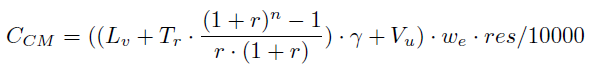where Lv is a raster map with the land use value [currency/ha];
Tr is a raster map with the tributes [currency/ha];
Vu is a raster map with the value upper part of the soil [currency/ha];
r is a scalar with the interest rate (default value: 0.03);
n is the life of a hydropower plant [year] (default value: 30);
γ is a scalar (default value: 5/4);
we is a scalar with the average width excavation [m] (default value: 2);
res is a scalar with the raster resolution

The Vu raster map is computed with the formula: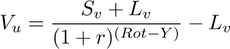where Sv is a raster map with the stumpage value [currency/ha];
Rot is a raster map with the value rotation period per land use type [year];
Y is a raster map with the average year [year]

The user can directly add the maps Lv, Tr, Sv, Rot and Y as inputs. Otherwise, the maps can be computed using the land use raster map and reclassifying the values with the module r.reclass. The program creates the reclassified maps if the user provides the input text files for each category (the input data is the path of the text file). Here is an example of a text file to create the landvalue raster map, the costs are in currency/ha:
```1 = 0 rocks, macerated, glaciers
2 = 0 urbanized areas, infrastructure
3 = 0 shores
4 = 0 waters
5 = 200 gardens
6 = 4000 mining areas
7 = 2000 agricultural areas
9 = 1000 areas with predominantly pastoral value
10 = 3000 forestry land```
Once the calculation is made, a new column with the compensation cost is added in the table of the input map with potential plants. A raster map with the compensation cost can also be computed, as well as a raster map with the value upper part of the soil (see Optional section).

- Excavation cost:

This cost concerns the works of digging to implement channels. It is calculated as followed: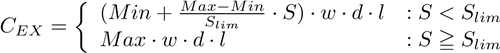where S is a raster map with the slope in [%];
Ψ is a raster map with values of minimum excavation costs [currency/mc];
Φ is a raster map with values of maximum excavation costs [currency/mc];
w is the width of the excavation [m] (default value: 2);
d is the depth of the excavation [m] (default value: 2);
l is the length of the excavation [m] which depends on the channels lengths

If the user hasn't got the raster maps Ψ and Φ, the latter can be computed from the land use raster map if the user provides a text file with the reclassification values (from land use value to excavation cost (min or max)). It is the same principle as the one explained above for landvalue, tributes, stumpage, rotation period per land use type and average age.

The user can choose to put a slope limit (Slim) [%] above which the cost will be equal to the maximum cost.

Then, a new column with the excavation cost is added in the table of the input map with potential plants. A raster map with excavation cost can also be computed (see Optional section).

- Electro-mechanical cost:

It is the cost of the electro-mechanical equipment which includes the turbine, the alternator and the regulator. It is a high percentage of a small hydropower plant budget (around 30% and 40% of the total sum).

We use the Aggidis et al. formula which calculates this cost thanks to the values of power and head:

CEM = γ * powerα * headβ + c
where power is the power of the plant [kW];
α is a power coefficient (default value: 0.56);
β is a head coefficient (default value: -0.112);
γ is a coefficient (default value: 15600);
c is a constant (default value: 0)
A new column with the electro-mechanical costs is added in the table of the input map with potential plants.

- Supply and installation cost for pipeline and electroline:

This is the sum of the supply and installation costs for the derivation channel, the penstock (both compose the pipeline) and the electroline which links the transformer near the turbine to the existing grid. The formula is the following: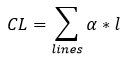where α is the supply and installation costs [currency/m] (default value for pipeline: 310 Euro/m, and for the electroline 250 Euro/m);
l is the length of the line [m], the pipeline length is found in the structure map and the electroline length is computed thanks to the grid vector map

- Power station cost:

It concerns the construction cost of the building composing the power station. It is considered as a percentage of the electro-mechanical cost:

CST = α * CEM
where α is as default 0.52

- Inlet cost:

It concerns the construction cost of the water intake structure. It is considered as a percentage of the electro-mechanical cost:

CIN = α *EM
where α is as default 0.38

All these costs define the Total cost:

Total cost = (CCM+CL+CEX+CEM+CST+CIN+CGRD)(1+α+β)
where CCM is the [currency];
CL is the Supply and installation cost for pipeline and electroline [currency];
CEX is the Excavation cost [currency];
CEM is the Electro-mechanical cost [currency];
CST is the Power station cost [currency];
CIN is the Inlet cost [currency];
CGRD is the Grid connection cost, (default is 50000 Euro), that includes the easement indemnity;
α is the factor to consider general expenses (default is 0.15);
β is the factor to consider hindrances expenses (default is 0.10)

α and β are factors which offset the underestimation of this total cost. Indeed, some other costs have to be taken into consideration but it's hard to make a general assessment because they are specific for each plant. Moreover, for each plant realization there are unexpected events (hindrances) which make the implementation more complex and expensive.

Then, the module calculates the maintenance cost per year, according to this formula :

maintenance = α * power1+β + c
where power is the power of the plant [kW];
α is a power coefficient (default value: 3871.2);
β is a power coefficient (default value: 0.45);
c is a constant (default value: 0)

The yearly revenue corresponds to the money gained selling all the electricity the plant produces in a year. It is simply calculated as the product of the power produced in a year by the price of the electricity (including some coefficients):

revenue = η * power * price * yh * α + c
where η is the efficiency of the electro-mechanical components (default value: 0.81);
power is the installed power of the plant [kW];
price is the energy price [currency/kW] (default value: 0.09 Euro/kW);
yh is the yearly operation hours of the plant [hours] (default value: 6500);
α is the coefficient to transform installed power to mean power (default value: 0.5);
c is a constant (default value: 0)

Finally, all these values allow to calculate the Net Present Value (NPV). It is the sum of the present values of incoming and outgoing cash flows over a period of time. It allows to know if there are profits so if the plant is feasible. It corresponds to: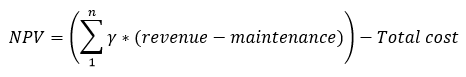where revenue is the yearly revenue value [currency/year];
maintenance is the yearly maintenance value [currency/year];
Total cost is the total cost of the plant [currency];
n the number of years of the plant [year] (default value: 30);
γ is a coefficient which assesses the cost of interest and amortization. It is defined as: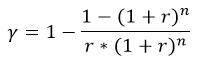where r is the interest rate (default value: 0.03)

More concretely, the program computes the following results:
- the input map with the structure of the plants has an updated table with the different costs of construction and implementation and their sum (tot_cost)

- the created output map shows the structure of the potential plants with a re-organized table. The latter doesn't make the difference between derivation channel and penstock. Each line gives the intake_id, plant_id, side (structures are computed on both sides of the river), power (kW), gross_head (m), discharge (m3/s), tot_cost (total cost for construction and implementation), yearly maintenance cost, yearly revenue, net present value (NPV) and max_NPV. The structure of potential plants is given for each side of the river, max_NPV is 'yes' for the side with the highest NPV and 'no' for the other side.

- the input map with the segments of the plants has an updated table with the total cost, yearly maintenance cost, yearly revenue and the net present value. The parameter "segment_basename" (in input column) allows to add a prefix at the column names in order to show the results for different cases in the same table without overwriting the columns.

- in the Optional section, there is the possibility to create three raster maps showing the compensation, excavation and upper part of the soil values

## EXAMPLE

This example is based on the case-study of Gesso and Vermenagna valleys in the Natural Park of the Maritime Alps, Piedmont, Italy.

Here in black you can see the input vector file techplants with the structure of the potential plants and the technical value of power (including head losses and efficiencies, computed by r.green.hydro.technical) computed by r.green.hydro.technical. The vector map with the segments of the river potentialplants is also visible in blue in this picture, as well as the vector map with the intakes and restitutions of the plants (in red).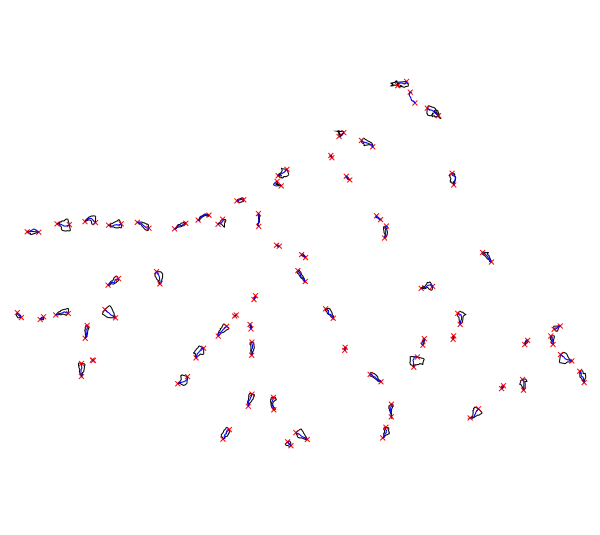structure of the potential plants

The following command updates the table of structplants and segplants adding the costs:

Notes:
The rules files for the reclassification needed for the following command can be created as shown in the section NOTES above for the rules_landvalue.
To create the other rule files this link might be helpful: r.category
The file techplants (input vector map with the structure of the plants) has to be created for example by r.green.hydro.technical before entering the following code.
```r.green.hydro.financial
plant=potentialplants
struct=techplants
landuse=landuse
rules_landvalue=/pathtothefile/landvalue.rules
rules_tributes=/pathtothefile/tributes.rules
rules_stumpage=/pathtothefile/stumpage.rules
rules_rotation=/pathtothefile/rotation.rules
rules_age=/pathtothefile/age.rules
slope=slope
rules_min_exc=/pathtothefile/excmin.rules
rules_max_exc=/pathtothefile/excmax.rules
electro=grid
output_struct=ecoplants
compensation=comp
excavation=exc
upper=upper```

It also creates four new raster maps (ecoplants, comp, exc and upper):
- ecoplants which shows the structure of the potential plants. The table contains these four columns (total cost, maintenance cost, revenue and NPV):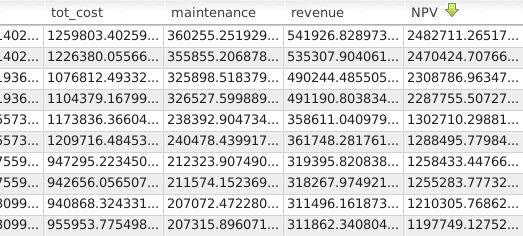table of the output raster map ecoplants

The same columns are added for the input map with the segments (potentialplants). In the table of the input map with the structure (techplants), the different costs which compose the total cost are added in columns (but not the four previous values).

- comp which shows the compensation values (in currency) for each land use: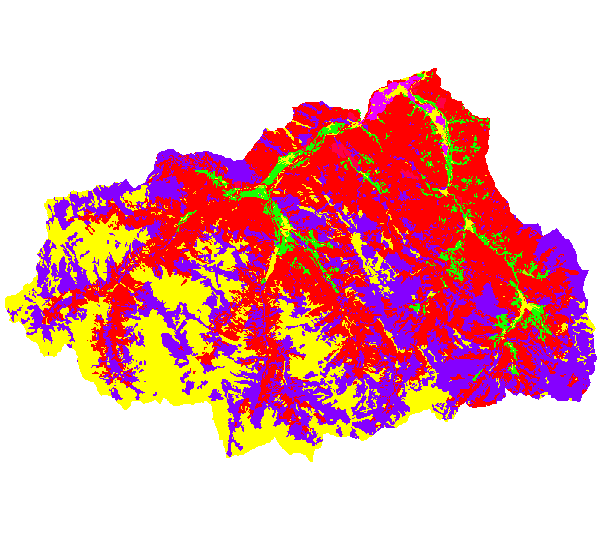output raster map with compensation values

- upper which shows the values of the upper part of the soil (in currency) for each land use

- exc which shows the excavation value (in currency) for each land use

These two last maps look like comp, but with their corresponding values.

r.green.hydro.discharge
r.green.hydro.delplants
r.green.hydro.theoretical
r.green.hydro.optimal
r.green.hydro.recommended
r.green.hydro.structure
r.green.hydro.technical

## REFERENCE

The costs of small-scale hydro power production: Impact on the development of existing potential, p.2635, Aggidis et Al, 2010

Text on expropriation for public utility D.P.R. n. 327/2001, updated in 2013

The excavation costs are found in the price list of the Piedmont Region for public works

The costs of intake and civil works are based on analysis of technical documents for mini-hydro projects in Italy

The calculation of the yearly maintenance cost is based on the report AEEG for the Polytechnic University of Milan

## AUTHORS

Pietro Zambelli (Eurac Research, Bolzano, Italy), Sandro Sacchelli (University of Florence, Italy), manual written by Julie Gros.

## SOURCE CODE

Available at: r.green.hydro.financial source code (history)

Latest change: Wed Mar 30 10:17:35 2022 in commit: 25b0a9981b66c443a1c1af1d5f26182c93268b45

© 2003-2022 GRASS Development Team, GRASS GIS 8.0.3dev Reference Manual